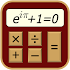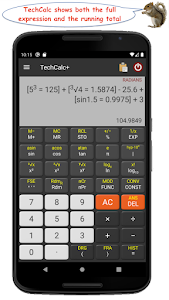# TechCalc+ Calculator v5.0.4 b345 (Paid)

### TechCalc+ Calculator / Screenshots### TechCalc+ Calculator / Description

TechCalc+ is the "Swiss Army Knife" of Scientific Calculators ... 44 Calculation Options + a Scientific Reference Section + the Periodic Table of the Elements!

Perfect for all aspects of scientific and engineering calculations in school university and throughout your career. Why not download it now and give it a try?

Modes included are:

★ Basic Mode - Algebraic and Reverse Polish Notation (RPN)
★ Scientific Mode - Algebraic and Reverse Polish Notation (RPN)
★ 64-bit Programmer Mode (Hex Oct Bin and Dec) - Algebraic and Reverse Polish Notation (RPN)
★ Graphing
★ Matrices
★ Complex Numbers (cartesian polar using Euler's identity)
★ Quick Formulas
★ Quick Converter
★ Time Calculator
★ Equation Solver
★ Calculus (Derivatives Definite Integrals Taylor Series Indefinite Integrals & Limits)
★ Financial

+ the Periodic Table of Elements!

Features include:

★ Powers & Roots
★ Logs and Antilogs
★ Factorial Modulus & Random Numbers functions
★ HCF LCM Prime factorization
★ Pol() & Rec() Functions
★ Permutations (nPr) and Combinations (nCr)
★ Statistics
★ Fractions Mode
★ A wide range of conversion categories and constants
★ 20 Memory Registers in each of the calculation modes
★ Detailed calculation history
★ Extensive Help and Reference
★ Highly customizable via the Settings

The reference section includes:

★ Physical Laws
★ Names in the Metric System
★ Mathematical Tables
★ Elementary & Linear Algebra
★ Trigonometric Identities
★ Differentiation & Integration Rules
★ Statistics Formulas
★ Vector Mathematics
★ ASCII IEEE 754 Fractional Bits Roman Numeral & Number Base Converters
★ pH Interpolation Body Mass Index (BMI) Percentage Color Proportion & Molecular Weight Calculators
★ Sigma & Pi Notation
★ Balancing Chemical Equations
★ Statistics (Grouped Data)
★ Numerical Sequences
★ Humidity Calculations
★ Boolean Algebra Calculator
★ Empirical Formula Calculator
★ Characteristics of an RLC Circuit
★ Feet and Inches Calculator
★ Aspect Ratio Calculator
★ Barometric Formula Calculator
★ Linear Regression Analysis (Simple Linear Regression; Multiple Linear Regression)
★ Bicycle Tire Pressure Calculator

Please email any questions that are not answered in the Help section.

### TechCalc+ Calculator / What's New in v5.0.4 b345 (Paid)

- ★ Untouched Paid apk with Original Hash Signature
★ Certificate MD5 digest: 4b13da30a6785cbf02c90911602b638d
★ No changes were applied
➡ Languages: Full Multi Languages
➡ CPU architectures: Universal
➡ Screen DPIs: 120dpi, 160dpi, 240dpi, 320dpi, 480dpi, 640dpi

@derrin

ver 5.0.4:
★ bug fixes, minor function enhancements and library updates